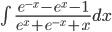WELCOME TO AP CALCULUS AB @ NMHS!

Built By Jacob Goldsmith (jgoldsmith@newarkunified.org) for AP Calculus AB @ Newark Memorial High School

Textbook references are to Larson 11th ed.

AP review references are to D&S 10th ed.

Note: all material ahead of the chapter we are currently working in is subject to revision

Introduction and items not connected to any specific chapter:

Syllabus

Chromebook info

Calculus BC page

AP join code: how to join, join codes

Chapter 1: limits

Video of practice test solutions - link

notes 1.2: introduction to limits

notes 1.3 (part 1): calculating advanced limits

notes 1.3:(part 2): trigonometric limits

notes 1.4: one-sided limits and continuity

notes 1.5: limits that equal positive or negative infinity

notes 3.5: limits as x approaches infinity and negative infinity

questions for assignment #8 (copied from AP review book)

quiz solutions

Chapter 2.1: derivatives

notes 2.1: introducing the difference quotient

notes 2.2: the power rule for derivatives and two trig. formulas qc solutions

notes 2.3: the product rule, the quotient rule, and four more trig. formulas (two classes) qc solutions #1 qc solutions #2

notes 2.4: the chain rule for derivatives (two classes) qc solutions

review hw #5 & bonus

quiz solutions

Chapter 2.2: applications of derivatives

notes 2.1: applications of differentiation

notes 2.2: physics, tangent lines & secant lines

notes 2.3 and 2.4: calculator tricks, instant rate of change and average rate of change, graphing calculator instructions (TI & Casio)

notes 2.5: implicit differentiation

notes 2.6: related rates, examples handout (2 days), assignment #7 worksheet

quiz solutions

benchmark quiz

Chapter 3.1: curve sketching

notes 3.1: absolute and relative maxima and minima

notes 3.3: increasing and decreasing functions

notes 3.4: inflection points and concave up/down functions

quiz solutions

AP practice

Chapter 3.2: advanced applications of derivatives

notes 3.2: Rolle’s Theorem and The Mean Value Theorem

notes 3.3 - 3.6: no notes, problems based on the relationships between f, f’, & f’’

notes 3.7: max/min problems

notes 3.9: differentials and local linear approximation

quiz solutions

AP practice

Chapter 4.1: introducing integration

notes 4.1: introducing integrals

notes 4.4: the definite integral

notes 4.3 and 4.4: surprise!

notes 4.5: integration by substitution (2 lessons), worksheet 300  (1-49)

quiz solutions

Chapter 4.2: applications of integration

Practice Test and notes, practice test solutions (note: change to #1b)

notes 4.3 and 4.4: area (repeated from previous notes)

notes (rectangular approximation): rectangular approximation and Riemann sums

notes 4.1: assorted applications of integration

notes 4.4: average value, the MVT for integrals, and the Fundamental Theorems of Calculus

quiz solutions

AP Practice

AP review book #3 & in-class test

Chapter 5.1: integration and differentiation continued

Practice Test  : change #19 toand formulas

log review worksheet

notes 5.1: derivatives involving y=ln(x)

notes 5.2: integrals involving ln(x) and additional trig. formulas

notes 5.4: (part 1): derivatives involving e

notes 5.4: (part 2): integrals involving e

notes 5.5: exponential and logarithmic equations with bases other than e (derivatives and integrals)

notes 5.7: inverse trig. derivatives

notes 5.8: inverse trig. integrals

AP Practice

AP review book #4 & in-class test

Chapter 5/6/7: advanced applications of integration

notes 7.2: the disc method

notes 7.1: area between two functions

notes 6.3: separable differential equations

notes 6.2: exponential growth and decay

notes 5.3: derivatives of inverse functions

notes 6.1: slope fields with separation of variables

Spring Break Assignment

Spring break assignment / bonus / schedule

AP review

AP Review: Physics

AP Review: Rates and Totals

AP Review: Separation of variables & slope fields

AP Review: Problems connecting f, f', f'', & integral(f)